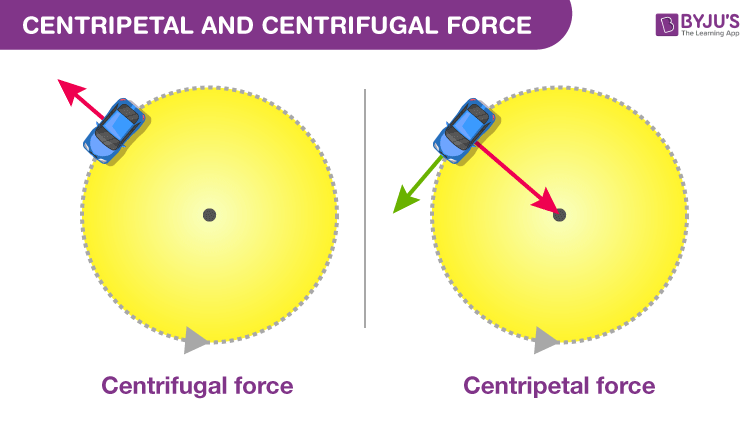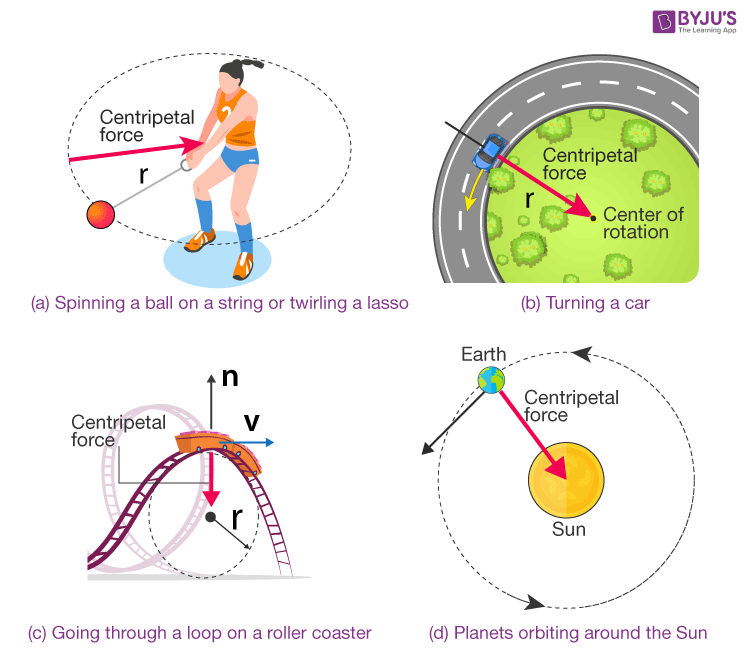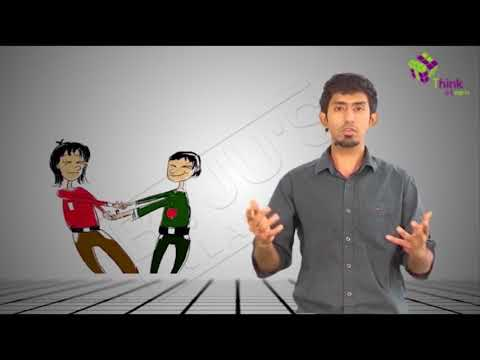# Centripetal And Centrifugal Force

Force is required to make an object move. Force acts differently on objects depending on the type of motion it exhibits. In the case of curvilinear motion, two types of force come into the picture, i.e., the centrifugal force and centripetal force. Centripetal force is the force acting towards the centre of the circular path. Whereas, the centrifugal force acts in a direction pointing away from the centre of the circle. In this article, let us discuss in detail what is centripetal force and centrifugal force.## Defining Centripetal Force

According to the centripetal force definition, it is defined as

Centripetal force is the component of force acting on an object in curvilinear motion which is directed toward the axis of rotation or centre of curvature.

The unit of centripetal force is Newton.

The centripetal force is always directed perpendicular to the direction of the displacement of the object. If an object accelerates according to the changes of velocity, then it can change either its speed or direction of motion. In simple terms, if any moving object in a circular path is constantly changing its direction means it is constantly accelerating. Using Newton’s second law of motion, if an object is travelling in a circular path, it is found that the centripetal force of an object moving in a circular path always acts towards the centre of the circle.

### Calculating Centripetal Force

The Centripetal Force Formula is given as the product of mass (in kg) and tangential velocity (in meters per second) squared, divided by the radius (in meters). Which implies that on doubling the tangential velocity, the centripetal force will be quadrupled. Mathematically it is written as:

 $F=ma_{c}=\frac{mv^{2}}{r}$

Where,

• F is the Centripetal force.
• ac is the Centripetal acceleration.
• m is the mass of the object.
• v is the speed or velocity of the object.

### Centripetal Force Examples in Daily Life

According to the centripetal force definition, we can say that it is the force that pulls or pushes an object toward the centre of a circle as it travels, causing angular or circular motion. Some examples of the Centripetal Force are given below.A few examples of Centripetal Force

• Spinning a ball on a string or twirling a lasso: Here the centripetal force is provided by the force of tension on the rope pulls the object in toward the centre.
• Turning a car: Here the centripetal force is provided by the frictional force between the ground and the wheels.
• Going through a loop on a roller coaster: The force is provided by the Normal Force as the seat or wall pushes you toward the centre.
• Planets orbiting around the Sun: Centripetal Force is provided by Gravity.
 Related Links on Centripetal Force Centripetal Acceleration Radial Acceleration Derivation of Centripetal Acceleration Central Force

## What is Centrifugal Force?

If an object moving in a circle and experiences an outward force than this force is called the centrifugal force. However, the force also depends on the mass of the object, the distance from the centre of the circle and also the speed of rotation. If the object has more mass, the force of the movement and the speed of the object will be greater. If the distance is far from the centre of the circle the force of the movement will be more.

Centrifugal force is a force that arises from the body’s inertia and appears to act on a body that is moving in a circular path which is directed away from the centre around which the body is moving.

Centrifugal force unit is Newton. The centrifugal force drives the object away from the centre. It is a fictitious force.

### How is Centrifugal Force calculated?

Centrifugal force formula is given as the negative product of mass (in kg) and tangential velocity (in meters per second) squared, divided by the radius (in meters). Which implies that on doubling the tangential velocity, the centripetal force will be quadrupled. Mathematically it is written as:

 $F_{c}=-\frac{mv^{2}}{r}$

Where,

• Fc is the Centrifugal force
• m is the mass of the object
• v is the velocity or speed of the object.

### Centrifugal Force Examples in Daily Life

Centrifugal Force acts on every object moving in a circular path when viewed from a rotating frame of reference. Some examples of Centrifugal Force are given below.

• Weight of an object at the poles and on the equator
• A bike making a turn.
• Vehicle driving around a curve
• Equatorial railway

## Why does the Moon keep going around the Earth?## Centripetal Force Vs Centrifugal Force

Check the table below to learn the detailed comparison between Centripetal and Centrifugal Force

Differences Between Centripetal And Centrifugal Force
Centrifugal Force
Centripetal Force
If an object moving in a circle and experiences an outward force then this force is called the centrifugal force. If the object travels at a uniform speed in a circular path it is called centripetal force.
The object has the direction along the centre of the circle from the centre approaching the object The object has the direction along the centre of the circle from the object approaching the centre.
Mud flying of a tire is one example of the centrifugal force. A satellite orbiting a planet is an example of the centripetal force.

## Frequently Asked Questions – FAQs

### What is Centripetal force?

Centripetal force is defined as the force required to keep a body moving in a circular path and directed towards the centre of a circle.

### Give examples of Centripetal force.

Following are the examples of centripetal force:

• The revolution of the moon around the earth.
• The spinning of the top.
• Rides in a roller coaster.

### What is the formula of centrifugal force?

Ans: $F_{c}=-\frac{mv^{2}}{r}$
Where,
• Fc is the centrifugal force
• m is the mass
• v is the speed

### What is the formula of centripetal force?

Ans: $F=\frac{mv^{2}}{r}$
Where,
• F is the centripetal force
• m is the mass
• v is the speed

### Compare Centrifugal force and centripetal force.

Centrifugal force is equal in magnitude and opposite in direction to the centripetal force.

Hope you have understood what is centripetal force in this article. To know more, visit BYJU’S – The Learning App.

Test Your Knowledge On Centripetal And Centrifugal Force!

What force applies to the planets that are revolving around sun. Centrifugal or centripetal ?

1. The centripetal force keeps the planets in orbit around the sun.

The sun’s gravity pulls on the planets just as Earth’s gravity that pulls down anything that is not held by up by some other force. All the planets would like to move in a straight line with constant speed, however, the sun’s gravity prevents it from doing so. Heavier object exerts stronger gravitational pull than the lighter ones, so the heavyweight in our solar system, the sun, exerts the stronger gravitational pull.

Now you might think if the sun is pulling the planet then why don’t they just fall into the sun and burn? Well, in addition to falling towards the sun, the planets are moving sideways too. Without the sideways motion, the planets would fall to the centre of the sun and without the pull toward the centre, it would go flying off in a straight line.

2. Moulabaksh

centripetal force

2. Vallinayagam

Cycle wheel rotation is example for centrifugal or centripetal force ?

1. Neither in itself. But an object attached to the rim of the wheel will experience a centripetal force preventing it being thrown off as the wheel rotates. Centripetal and centrifugal force is equal and opposite but the latter does not actually exist unless a centripetal force is being applied by a retaining cord or gravity or containing rim outside the object.